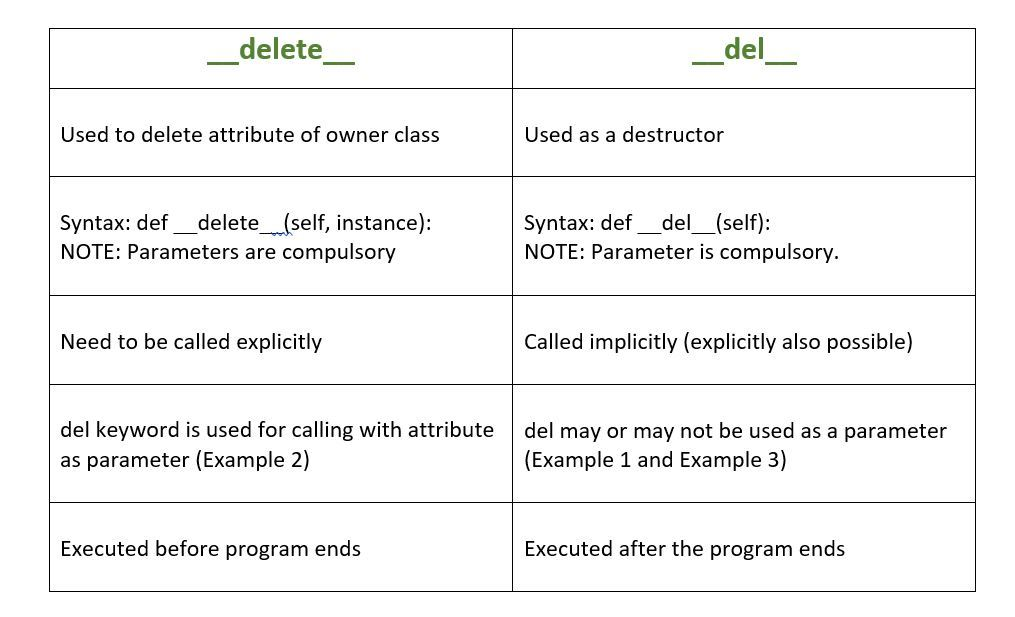# Python : __delete__ vs __del__

Both `__delete__` and `__del__` are dunder or magic methods in Python. Dunder or magic methods in Python are the methods having two prefix and suffix underscores in the method name. Dunder here means “Double Under (Underscores)”. These are commonly used for operator overloading.

#### __del__

`__del__` is a destructor method which is called as soon as all references of the object are deleted i.e when an object is garbage collected.

Syntax:

```def __del__(self):
body of destructor
.
.
```

Example: Here is the simple example of destructor. By using del keyword we deleted the all references of object ‘obj’, therefore destructor invoked automatically.

 `# Python program to demonstrate ` `# __del__ ` ` `  ` `  `class` `Example:  ` `   `  `    ``# Initializing ` `    ``def` `__init__(``self``):  ` `        ``print``(``"Example Instance."``) ` ` `  `    ``# Calling destructor ` `    ``def` `__del__(``self``):  ` `        ``print``(``"Destructor called, Example deleted."``)  ` `   `  `obj ``=` `Example()  ` `del` `obj  `

Output:

```Example Instance.
Destructor called, Example deleted.
```

Note : The destructor was called after the program ended or when all the references to object are deleted i.e when the reference count becomes zero, not when object went out of scope.

#### __delete__

`__delete__` is used to delete the attribute of an instance i.e removing the value of attribute present in the owner class for an instance.

Note: This method only deletes the attribute which is a descriptor.

Syntax:

```def __delete__(self, instance):
body of delete
.
.
```

Example:

 `# Python program to demonstrate ` `# __delete__ ` ` `  ` `  `class` `Example(``object``): ` ` `  `    ``# Initializing ` `    ``def` `__init__(``self``): ` `        ``print``(``"Example Instance."``) ` ` `  `    ``# Calling __delete__ ` `    ``def` `__delete__(``self``, instance): ` `        ``print` `(``"Deleted in Example object."``) ` ` `  ` `  `# Creating object of Example ` `# class as an descriptor attribute ` `# of this class ` `class` `Foo(``object``): ` `    ``exp ``=` `Example() ` ` `  `# Driver's code ` `f ``=` `Foo() ` `del` `f.exp `

Output:

```Example Instance.
Deleted in Example object.
```

#### Difference between __delete and __del__Example: A combine example of `__del__` and` __delete__`.

 `# Python program to demonstrate ` `# __del__ and __delete__ ` ` `  ` `  `class` `Example(``object``): ` ` `  `    ``# Initializing ` `    ``def` `__init__(``self``): ` `        ``self``.value ``=` `'' ` ` `  `    ``# deletes an attribute ` `    ``def` `__delete__(``self``, instance): ` `        ``print` `(``"Inside __delete__"``) ` `         `  `    ``# Destructor ` `    ``def` `__del__(``self``): ` `        ``print``(``"Inside __del__"``) ` `     `  `     `  `class` `Foo(``object``): ` `    ``exp ``=` `Example() ` ` `  `# Driver's code ` `f ``=` `Foo() ` `del` `f.exp `

Output:

```Inside __delete__
Inside __del__
```

My Personal Notes arrow_drop_upCheck out this Author's contributed articles.

If you like GeeksforGeeks and would like to contribute, you can also write an article using contribute.geeksforgeeks.org or mail your article to contribute@geeksforgeeks.org. See your article appearing on the GeeksforGeeks main page and help other Geeks.

Please Improve this article if you find anything incorrect by clicking on the "Improve Article" button below.

Article Tags :

Be the First to upvote.

Please write to us at contribute@geeksforgeeks.org to report any issue with the above content.